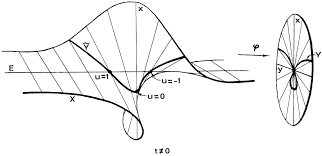# Matthew Weaver - ResearchMy research interests lie in commutative algebra and I am particularly interested in its connections and applications to algebraic geometry. Shown above is the blow-up of the plane at the origin showing how the node singularity of a curve can be resolved. This construction is a classical tool within birational geometry and the study of singularities and can be generalized to produce the blow-up of any variety along a given subvariety (although the picture gets much harder to draw!). My research centers around these objects, but I study them from the algebraic point of view, namely through the so-called blow-up algebras.

Given an ideal $$I=(\alpha_1,\ldots,\alpha_n)$$ of a commutative Noetherian ring $$R$$, the Rees algebra is the graded ring $$\mathcal{R}(I) = R[\alpha_1 t,\ldots,\alpha_n t] \cong \bigoplus_{i=0}^\infty I^it^i$$ viewed as a subring of $$R[t]$$. Although seemingly unrelated, this algebraic object is deeply connected to geometry as this is the homogeneous coordinate ring of the blow-up of $${\rm Spec}(R)$$ along the subscheme $$V(I)$$. The most lucrative way to study these rings is through their defining ideals. More precisely, there is a natural surjective map of $$R$$-algebras $$\Psi:\,R[T_1,\ldots,T_n] \rightarrow \mathcal{R}(I)$$. As this is an epimorphism, one needs only to understand $$\mathcal{J} = {\rm ker}\, \Psi$$, the defining ideal of $$\mathcal{R}(I)$$, in order to understand $$\mathcal{R}(I)$$.

This is much easier said than done as $$\mathcal{J}$$ encodes all of the polynomial relations on the generators of $$I$$. Certainly you could write some of these equations down, but how could you ever be sure you found all of them? Even more problematic, depending on the ring $$R$$, there could be additional relations one would need to consider! One would often like a minimal generating set, of defining equations, of $$\mathcal{J}$$ and obtaining these equations has been my current research focus.

I am particularly interested in how techniques from other branches of mathematics can be used to produce these equations. I have had success using algorithms and other computational techniques studying the defining ideal of $$\mathcal{R}(I)$$ for notable classes of ideals in hypersurface rings. These equations can also be described in terms of differential operators and these techniques can be applied to study the defining ideals of Rees algebras of modules as well.

Recent Materials

Below you can find some of my recent preprints.

• On Rees algebras of ideals and modules over hypersurface rings, (PDF, arXiv)
• The equations defining Rees algebras of Gorenstein ideals of codimension three in hypersurface rings, ( in prepration)

If you'd like to learn more about my work, here is a brief "elevator pitch" video where I give an overview of my research interests.